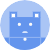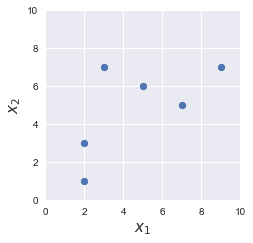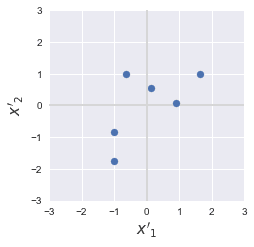search
Search
Publish
Guest 0reps
Thanks for the thanks!
close
Guides
Cancel
Post
account_circle
Profile
exit_to_app
Sign out
search
keyboard_voice
close
Searching Tips
Search for a recipe: "Creating a table in MySQL"
Search for an API documentation: "@append"
Search for code: "!dataframe"
Apply a tag filter: "#python"
Useful Shortcuts
/ to open search panel
Esc to close search panel
to navigate between search results
d to clear all current filters
Enter to expand content previewDoc SearchCode Search BetaSORRY NOTHING FOUND!
mic
Start speaking...Voice search is only supported in Safari and Chrome.
Shrink
Navigate to
A
A
share
thumb_up_alt
bookmark
arrow_backShareTwitterFacebook
Guides
thumb_up
0
thumb_down
0
chat_bubble_outline
0
auto_stories new
settings

# Gentle Introduction to Feature Scaling

Machine Learning
chevron_right
Feature Engineering
schedule Mar 26, 2022
Last updated
local_offer Machine LearningPython
Tags

# Why is scaling needed?

Many machine learning algorithms can benefit from feature scaling. Here are some of the cases when scaling can help:

• algorithms that make use of distance metrics will be heavily skewed by the magnitude of the features. For instance, the k-nearest neighbour algorithm utilises Euclidean distance, and whether a feature value is in grams (5000 grams) or in kilograms (5 kg) will largely dictate the distance.

• algorithms that compute variance. For instance, principle component analysis selects features with the highest variance, and hence, features with higher order of magnitude will always be erroneously selected.

• algorithms that make use of gradient descent. In practice, gradient descent converges much faster if feature values are smaller. This means that feature scaling is beneficial for algorithms such as linear regression that may use gradient descent for optimisation.

# Scaling techniques

There are several ways to perform feature scaling. Some of the common ways are as follows:

1. Standardisation

2. Mean Normalisation

3. Min-max Scaling

## Standardisation

The formula for standardisation, which is also known as Z-score normalisation, is as follows:

$$\begin{equation}\label{eq:vgKhK1G7HFMsCuP90sf} x'=\frac{x-\bar{x}}{\sigma} \end{equation}$$

Where:

• $x'$ is the scaled value of the feature

• $x$ is the original value of the feature

• $\bar{x}$ is the mean of all values in the feature

• $\sigma$ is the standard deviance of all values in the feature. We often just stick with the biased estimate in machine learning - check out the example below for clarification.

The standardised features have a mean of $0$ and a standard deviation of $1$. Let us now prove this claim.

### Mathematical proof that the mean is zero and standard deviation is one

Suppose we standardise some raw feature value $x_i$. The mean of the standardised feature values $\bar{x}'$ is:

\begin{align*} \bar{x}' &=\frac{1}{n}\sum^n_{i=1}(x'_i)\\ &=\frac{1}{n}\sum^n_{i=1}\frac{(x_i-\bar{x})}{\sigma}\\ &=\frac{1}{n\sigma}\Big[\Big(\sum^n_{i=1}x_i\Big)-n\bar{x}\Big]\\ &=\Big(\frac{1}{\sigma}\cdot\frac{1}{n}\sum^n_{i=1}x_i\Big)-\frac{\bar{x}}{\sigma}\\ &=\frac{\bar{x}}{\sigma}-\frac{\bar{x}}{\sigma}\\ &=0\\ \end{align*}

Next, the variance of the standardised feature values $\sigma^2_{x'}$ is:

\begin{align*} \sigma^2_{x'}&=\frac{1}{n}\sum^n_{i=1}({x'_i}-{\bar{x}'})^2\\ &=\frac{1}{n}\sum^n_{i=1}\left(\frac{x_i-\bar{x}}{\sigma_x}-0\right)^2\\ &=\frac{1}{n\cdot\sigma^2_x}\sum^n_{i=1}\left(x-\bar{x}\right)^2\\ &=\frac{1}{\sigma^2_x}\cdot{}\frac{1}{n}\sum^n_{i=1}\left(x-\bar{x}\right)^2\\ &=\frac{1}{\sigma^2_x}\cdot{}\sigma^2_x\\ &=1\\ \end{align*}

Since the variance $\sigma^2_{x'}$ is $1$, the standard deviation $\sigma_{x'}$ is of course also $1$.

### Simple example of standardising

Suppose we had the following dataset with one feature:

$x_1$

1

5

2

3

3

7

Let's standardise feature $x_1$. To do so, we need to compute the mean and variance of $x_1$ - let's start with the mean:

\begin{align*} \bar{x}_1&=\frac{1}{3}(5+3+7)\\ &=5 \end{align*}

Next, let's compute the standard deviation of $x_1$:

\begin{align*} \sigma_1&=\sqrt{\frac{1}{3}\sum^3_{i=1}(x_i-\bar{x})^2}\\ &=\sqrt{\frac{1}{3}\Big[(5-5)^2+(3-5)^2+(7-5)^2\Big]}\\ &\approx1.63 \end{align*}

Great, we now have everything we need to perform standardisation on $x_1$!

WARNING

In statistics, we often compute the unbiased estimate of the standard deviation, that is we divide by $n-1$ instead of $n$:

$$\sigma=\sqrt{\frac{1}{n-1}\sum^n_{i=1}(x_i-\bar{x})^2}\\$$

When performing standardisation, we almost never use this version because we are only interested in making the mean of the feature 0 and the standard deviation 1.

For notational convenience, let's express the scaled feature as $d$ instead of $x'$. For each value in $x_1$, we need to perform:

\begin{align*} d^{(i)}_1&=\frac{x_1^{(i)}-\bar{x}_1}{\sigma_1}\\ &=\frac{x_1^{(i)}-5}{1.63} \end{align*}

Where:

• $d^{(i)}_1$ is the scaled value of the $i$-th value in feature $x_1$.

• $x^{(i)}_1$ is the original $i$-th value in feature $x_1$.

For instance, the first scaled feature value is:

\begin{align*} d^{(1)}_1&=\frac{x_1^{(1)}-5}{1.63}\\ &=\frac{5-5}{1.63}\\ &=0 \end{align*}

And for the second is:

\begin{align*} d^{(2)}_1&=\frac{x_1^{(2)}-5}{1.63}\\ &=\frac{3-5}{1.63}\\ &\approx-1.23 \end{align*}

And so on.

The scaled values of $x_1$ are summarised below:

$d_1$

1

0

2

-1.23

3

1.23

If there were other features, then you would need to perform these exact same steps for every single one of those features.

### The pattern of the data points are preserved

The overall layout of our data points should look the same even after performing standardisation. To demonstrate, here is a side-by-side comparison of a before and after:

Before

AfterCan you see how the overall pattern of our data points is preserved? The key difference though is that the standardised data points are centered around the origin with an overall spread of one.

## Mean normalisation

The formula for mean normalisation is as follows:

$$x'=\frac{x-\bar{x}}{x_{max}-x_{min}}$$

Where:

• $x'$ is the scaled value of the feature

• $x$ is the original value of the feature

• $\bar{x}$ is the mean of all values in the feature

• $x_{min}$ is the smallest value of the feature

• $x_{max}$ is the largest value of the feature

The denominator, $x_{max}-x_{min}$, is essentially the range of the feature. By applying this transformation, we can ensure that the following property holds:

• all values in the scaled feature $x'$ lies between $-1$ and $1$

• the mean of the scaled feature $x'$ is $0$.

WARNING

In practice, mean normalisation is not often used. Instead, either standardisation or min-max scaling is used.

## Min-max scaling

The formula for min-max scaling is very similar to that for mean normalisation:

$$x'=\frac{x-x_{min}}{x_{max}-x_{min}}$$

After the transformation, we can guarantee that all the values in the scaled feature $x'$ lie between $0$ and $1$.

# Misconceptions

## Scaling the dependent variable

There is no need to perform scaling for dependent variables since the purpose of feature scaling is to ensure that all features are treated equally by our model. This is the reason why "feature scaling" specifically contains the word "feature"!

## Scaling training and testing data separately

We should not scale training and testing data using separate scaling parameters. For instance, suppose we want to scale our dataset, which has been partitioned into training and testing sets, using mean normalisation. The scaling parameters for mean normalisation of a particular feature are its:

• mean $x'$

• minimum $x_{min}$

• maximum $x_{max}$

The correct way of performing mean normalisation would be to compute these parameters using only the training data, and then instead of re-computing the parameters separately for the testing data, we reuse the parameters we obtained for the training data. Therefore, we need to ensure that we store the parameters for later use.

The reason for this is that feature scaling should be interpreted as part of the model itself. In the same way the model parameter values obtained after training should be used to process the testing data, the same parameter values (e.g. $x_{min}$) obtained for feature scaling should be used for the testing data.

## Best scaling technique

There is no single best scaling technique. That said, either standardisation or min-max scaling is often used in practice instead of mean normalisation. One could also compare the performance between the two to decide which to ultimately choose.

## Normalisation and standardisation

The terms normalisation and standardisation are often confused. In machine learning, normalisation typically refers to min-max scaling (scaled features lie between $0$ and $1$), while standardisation refers to the case when the scaled features have a mean of $0$ and a variance of $1$.

# Performing feature scaling on Python

## Standardisation

To perform standardisation, use the StandardScaler module from the sklearn library:

 import numpy as npfrom sklearn.preprocessing import StandardScaler# 4 samples/observations and 2 featuresX = np.array([[5,3],[4,2],[1,6],[3,1]])# Fit and transform the datascaler = StandardScaler()scaled_X = scaler.fit_transform(X)scaled_X array([[ 1.18321596, 0. ], [ 0.50709255, -0.53452248], [-1.52127766, 1.60356745], [-0.16903085, -1.06904497]]) 

Note that a new array is returned and the original X is unaffected.

We can confirm that the mean of the features of scaled_X is 0:

 np.mean(scaled_X, axis=0) # axis=0 means that we compute the mean for each column array([-1.38777878e-17, 0.00000000e+00]) 

Note that the reason the mean for the first column is not exactly 0 is due to the nature of floating numbers.

To confirm that the variance of the features of scaled_X is 1:

 np.var(scaled_X, axis=0) # axis=0 means that we compute the variance for each column (feature) array([1., 1.]) 

## Min-max scaling

To perform min-max scaling, use the MinMaxScaler module from the sklearn library:

 import numpy as npfrom sklearn.preprocessing import MinMaxScaler# 4 samples/observations and 2 featuresX = np.array([[5,3],[4,2],[1,6],[3,1]])# Fit and transform the datascaler = MinMaxScaler()scaled_X = scaler.fit_transform(X)scaled_X array([[1. , 0.4 ], [0.75, 0.2 ], [0. , 1. ], [0.5 , 0. ]]) 

Note the following:

• a new array is returned and the original X is kept intact.

• the column values of scaled_X now range from $0$ to $1$.